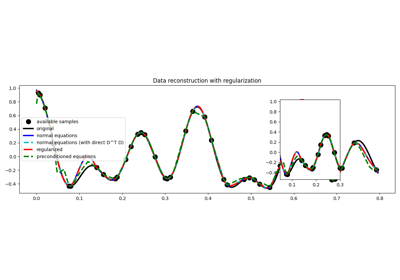# pylops.optimization.sparsity.spgl1#

pylops.optimization.sparsity.spgl1(Op, y, x0=None, SOp=None, tau=0.0, sigma=0.0, show=False, **kwargs_spgl1)[source]#

Solve a constrained system of equations given the operator `Op` and a sparsyfing transform `SOp` aiming to retrive a model that is sparse in the sparsyfing domain.

This is a simple wrapper to `spgl1.spgl1` which is a porting of the well-known SPGL1 MATLAB solver into Python. In order to be able to use this solver you need to have installed the `spgl1` library.

Parameters
Op`pylops.LinearOperator`

Operator to invert

y`numpy.ndarray`

Data

x0`numpy.ndarray`, optional

Initial guess

SOp`pylops.LinearOperator`, optional

Sparsifying transform

tau`float`, optional

Non-negative LASSO scalar. If different from `0`, SPGL1 will solve LASSO problem

sigma`list`, optional

BPDN scalar. If different from `0`, SPGL1 will solve BPDN problem

show`bool`, optional

Display iterations log

**kwargs_spgl1

Arbitrary keyword arguments for `spgl1.spgl1` solver

Returns
xinv`numpy.ndarray`

Inverted model in original domain.

pinv`numpy.ndarray`

Inverted model in sparse domain.

info`dict`

Dictionary with the following information:

• `tau`, final value of tau (see sigma above)

• `rnorm`, two-norm of the optimal residual

• `rgap`, relative duality gap (an optimality measure)

• `gnorm`, Lagrange multiplier of (LASSO)

• `stat`, final status of solver
• `1`: found a BPDN solution,

• `2`: found a BP solution; exit based on small gradient,

• `3`: found a BP solution; exit based on small residual,

• `4`: found a LASSO solution,

• `5`: error, too many iterations,

• `6`: error, linesearch failed,

• `7`: error, found suboptimal BP solution,

• `8`: error, too many matrix-vector products.

• `niters`, number of iterations

• `nProdA`, number of multiplications with A

• `nProdAt`, number of multiplications with A’

• `n_newton`, number of Newton steps

• `time_project`, projection time (seconds)

• `time_matprod`, matrix-vector multiplications time (seconds)

• `time_total`, total solution time (seconds)

• `niters_lsqr`, number of lsqr iterations (if `subspace_min=True`)

• `xnorm1`, L1-norm model solution history through iterations

• `rnorm2`, L2-norm residual history through iterations

• `lambdaa`, Lagrange multiplier history through iterations

Raises
ModuleNotFoundError

If the `spgl1` library is not installed

Notes

## Examples using `pylops.optimization.sparsity.spgl1`#03. Solvers

03. Solvers